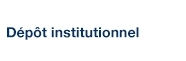# Dehn fillings of knot manifolds containing essential once-punctured tori

Boyer, Steven; Gordon, C. McA. et Zhang, X. (2013). « Dehn fillings of knot manifolds containing essential once-punctured tori ». Transactions of the American Mathematical Society, 366(1), pp. 341-393.

Fichier(s) associé(s) à ce document :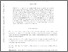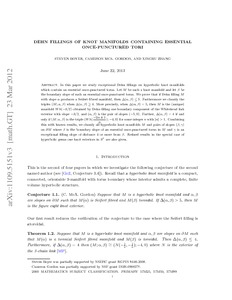Prévisualisation
PDF
Télécharger (550kB)

## Résumé

In this paper we study exceptional Dehn fillings on hyperbolic knot manifolds which contain an essential once-punctured torus. Let M be such a knot manifold and let β be the boundary slope of such an essential once-punctured torus. We prove that if Dehn filling M with slope α produces a Seifert fibred manifold, then Δ(α, β) ≤ 5. Furthermore we classify the triples (M; α, β) when Δ(α, β) ≥ 4. More precisely, when Δ(α, β) = 5, then M is the (unique) manifold W h(-3/2) obtained by Dehn filling one boundary component of the Whitehead link exterior with slope -3/2, and (α, β) is the pair of slopes (-5, 0). Further, Δ(α, β) = 4 if and only if (M; α, β) is the triple (W h(-2n ± 1/n); -4, 0) for some integer n with n > 1. Combining this with known results, we classify all hyperbolic knot manifolds M and pairs of slopes (β, γ) on ∂M where β is the boundary slope of an essential once-punctured torus in M and γ is an exceptional filling slope of distance 4 or more from β. Refined results in the special case of hyperbolic genus one knot exteriors in S3 are also given.

Type: Article de revue scientifique Dehn fillings, hyperbolic knot manifolds Faculté des sciences > Département de mathématiques Steven P. Boyer 27 avr. 2016 13:40 19 mai 2016 18:00 http://archipel.uqam.ca/id/eprint/8322

## Statistiques

Voir les statistiques sur cinq ans...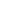RECHERCHER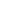PARCOURIR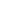LIBRE ACCÈS